﻿ 导航终端多模宽带微带天线馈电网络设计 Design Feed Network for Multi-Mode Broadband Microstrip Antenna of Navigation Terminal

Journal of Antennas
Vol. 07  No. 02 ( 2018 ), Article ID: 27279 , 8 pages
10.12677/JA.2018.72002

Pingchuan Zhang*, Hongxing Sun, Sijie Wang, Jiayi Zhu, Peixin Qu

School of Information Engineering, Henan Institute of Science and Technology, Xinxiang Henan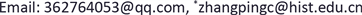Received: Sep. 29th, 2018; accepted: Oct. 19th, 2018; published: Oct. 26th, 2018ABSTRACT

In order to meet the demand of improving navigation service quality through receiving signals from the four GNSS systems, a four-feed network is designed and implemented for the multi-mode broadband microstrip antenna of navigation terminal. The right-handed polarization is obtained by applying 90-degree phase difference and other amplitude excitation signals at the port 1 to port 4 successively, improving the purity of circular polarization, and obtaining the circular polarization bandwidth that meets the design requirements. The comprehensive simulation using Designer and HFSS software shows that the antenna has good electrical performance and radiation characteristics within the frequency range of the four navigation systems. The vertex axial ratio is less than 3 dB; the impedance bandwidth is 45%; and the gain is greater than 6 dB; and the echo loss S11 is less than −10 dB, which ensures the accuracy needed in multi-mode navigation.1. 引言

2. 多模宽带微带天线贴片设计

$W=\frac{0.707C}{{f}_{r}}{\left({\epsilon }_{r}+1\right)}^{-0.5}$ (1)

$\Delta L=0.412\frac{\left({\epsilon }_{r}+0.3\right)\left(\frac{W}{h}+0.264\right)}{\left({\epsilon }_{r}-0.258\right)\left(\frac{W}{h}+0.8\right)}$ (2)

$L={\lambda }_{g}/2-\Delta L$ (3)

${\epsilon }_{e1}=0.5\left({\epsilon }_{r}+1\right)+0.5\left({\epsilon }_{r}-1\right){\left(1+\frac{10h}{W}\right)}^{-0.5}$ (4)

${\epsilon }_{e}=\frac{{\epsilon }_{e1}{\epsilon }_{e2}\left(h+hl\right)}{{\epsilon }_{e2}h+{\epsilon }_{e1}hl}$ (5)

${\lambda }_{g}=\frac{{\lambda }_{0}}{\sqrt{{\epsilon }_{e}}}=\frac{c}{2{f}_{r}\sqrt{{\epsilon }_{e}}}$ (6)

3. 馈电网络设计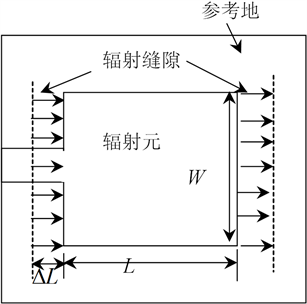Figure 1. Structure of the rectangular microstrip patch antenna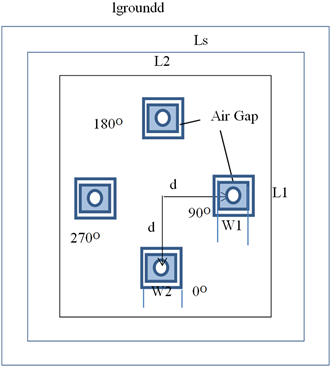Figure 2. Birdview diagram of four-feed structure of rectangular patchFigure 3. Lateral view of four feed of multimode microstrip broadband antenna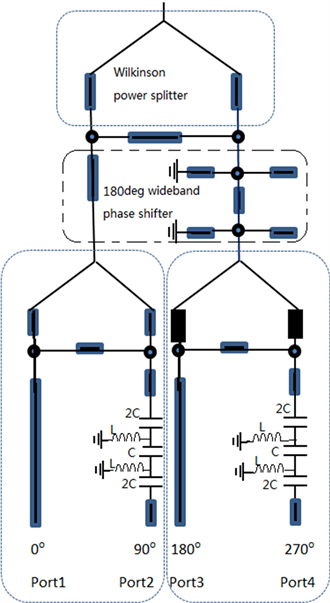Figure 4. Phase shifter of four-feed points network

4. 仿真及结果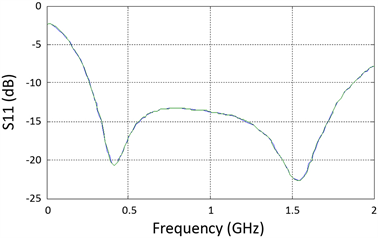Figure 5. Simulation results of S11 fed by multi-mode microstrip antenna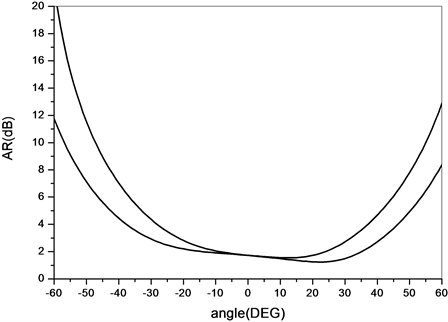Figure 6. The axial ratio diagram of 1561 MHz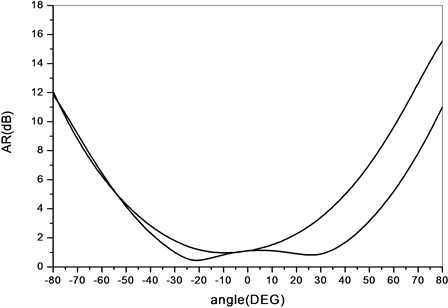Figure 7. The axial ratio diagram of 1561 MHz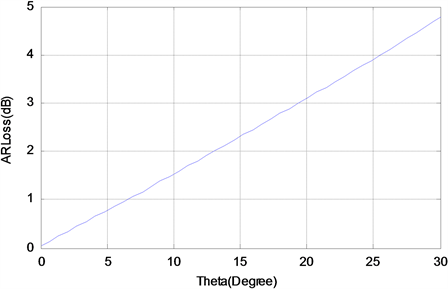Figure 8. Phase error versus axial loss

5. 结语

Design Feed Network for Multi-Mode Broadband Microstrip Antenna of Navigation Terminal[J]. 天线学报, 2018, 07(02): 9-16. https://doi.org/10.12677/JA.2018.72002

1. 1. 陶金锋. 多模卫星导航系统天线设计[D]: [硕士学位论文]. 北京: 北京邮电大学, 2011.

2. 2. 付灿, 李民权, 金秀梅. 应用于多模卫星定位导航系统的宽带天线设计[J]. 合肥工业大学学报(自然科学版), 2011(3): 391-394.

3. 3. 唐洪军, 郑先宝, 张书文. 一种短路环状叠层宽带GNSS天线设计[J]. 中国科技信息, 2016(18): 80-81.

4. 4. 田印炯. 多模卫星导航终端天线的研究[D]: [硕士学位论文]. 杭州: 杭州电子科技大学, 2014.

5. 5. 刘宝国, 徐凌伟, 张浩, 等. 北斗导航系统中天线阵的选择研究[J]. 信息网络安全, 2015(1): 12-15.

6. 6. Fu, L., Liu, Z.-G., Cao, J., et al. (2014) Design of a Multi-System Circularly-Polarized Square Microstrip Patch Antenna for GNSS Navigation Devices. Proceedings of 2014 3rd Asia-Pacific Conference on Antennas and Propagation (APCAP 2014), Harbin, 26-29 July 2014, 476-479.

7. 7. Shin, J.Y., Park, C.H. and Woo, J.M. (2018) Design of Microstrip Antenna for Satellite Navigation System Jamming. Journal of Positioning, Navigation, and Timing, 7, 37-42.

8. 8. 李运志, 袁子伦, 李军. 多模GNSS天线技术研究与设计[J]. 中国科学: 物理学力学, 天文学, 2011(5): 564-567.

9. 9. 商锋, 刘一江. 多模导航系统的接收天线的设计[J]. 西安邮电大学学报, 2014, 19(6): 62-65.

10. 10. 曾慧灵. 宽带多模天线设计研究[D]: [硕士学位论文]. 成都: 电子科技大学, 2017.

11. 11. Xu, Z., Ma, H., Wang, J., et al. (2014) Dual-Band Du-al-Polarized Microstrip Antenna for Compass Navigation Satellite System. Progress in Electromagnetics Research C, 46, 25-30.

12. 12. 张更, 王威, 霍小宁, 王煊, 杨绰, 金文, 王晓飞. 小型化四馈点多模导航天线设计[J]. 空间电子技术, 2017(6): 85-88.

13. 13. Yuan, H.Y., Zhang, J.Q., Qu, S.B., et al. (2012) Dual-Band Dual-Polarized Microstrip An-tenna for Compass Navigation Satellite System. Progress in Electromagnetics Research C, 30, 213-223.
https://doi.org/10.2528/PIERC12041714

14. 14. 宋跃, 刘岚, 韩国栋. 北斗多模卫星导航天线设计[J]. 电子科技, 2013(4): 137-139.

15. 15. 胡少青. 多模全球卫星导航系统天线研究[D]: [硕士学位论文]. 成都: 电子科技大学, 2016.

16. 16. Ye, S., Martynyuk, D., Vasylenko, O., et al. (2015) Novel Microstrip Antenna Array for Anti-Jam Satellite Navigation System. Radioelectronics and Communications Systems, 58, 97-106.
https://doi.org/10.3103/S0735272715030012